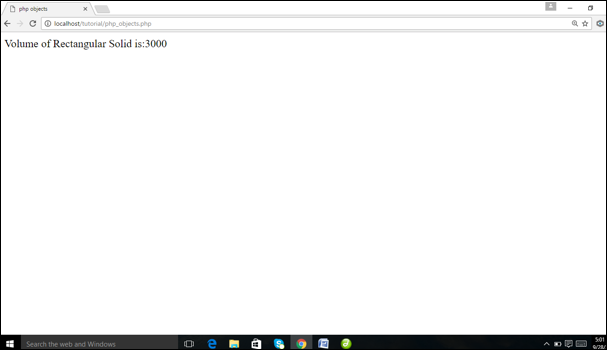PHP Object Oriented Programming | Coding Tag# PHP Object Oriented Programming

3642

Programming, in simple words, means giving instructions to a computer to process the data and provides the required output. There are mainly two programming approaches, Procedure Oriented Programming (POP) and Object Oriented Programming (OOP).

In procedure Oriented Programming, you first break the problem into smaller sections of code and then solved each section separately. Later on, all the solved sections of the program are integrated to solve the entire problem. Each small section of the code is written within a block of code, called a method. You can call one method from another. Therefore, these methods are dependent on each other. As a result, re-usability of the applications becomes difficult.

The concept of object-oriented programming revolves entirely around objects and their interaction to design applications and computer programs.

The concept of object-oriented programming has been introduced to overcome the difficulty of limited or no re-usability of code. It also adopts the approach of combining the independent object, which interact with each other to solve the overall problems.

Amongst these two approaches, OOP is considered as the better one, since it follows a model related to real world objects.

There is various advantage of using object-oriented programming.

RE-USABILITY OF CODE: In POP methods are dependent to each other, but if you will use OOP concept greater re-usability of code maintains in a programming.

EASY TO HANDLE:  Object-oriented programming techniques are easier to handle. We used class and object concept in OOP.

GREAT LEVEL OF ABSTRACTION:  In OOP we used approach of combining the independent object, which interact with each other to solve the overall problems and execute the business logic of the program.

How OOP Work?

Basic concept of object oriented programming in PHP then it is classes and objects.
CLASS is very easy to defined and create in PHP. We used tag class.

For Example:

In this example we create a class Rectangular_Solid_Volume. We create a function calculateVolume()  to calculate the volume of rectangular solid. We used the formula length * width * height.

```class Rectangular_Solid_Volume

{
var \$length;
var \$width;
var \$height;
function calculateVolume()
{
return (\$this->length*\$this->width*\$this->height);
}
}
// Now we create an object of class using new keyword.
\$RS_volume = new Rectangular_Solid_Volume();
// Now set the value of length, width, height and calculates volume of // rectangular solid.
\$RS_volume->length = 20;
\$RS_volume->width =10;
\$RS_volume->height = 15;
// call function and print volume
echo "Volume of Rectangular Solid is:".  \$RS_volume->calculateVolume();```
Result:Share:## SEMRush FREE Trial – PRO Account for 14 Days

FREE Pro Account worth \$99.95 for 14 Days.

## Big Hosting Days

Upto 75@ OFF on Web Hosting

## Get a .COM for just \$6.98

Secure Domain for a Mini Price